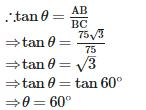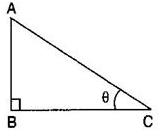Courses

# RD Sharma Test: Some Applications of Trigonometry

## 25 Questions MCQ Test Mathematics (Maths) Class 10 | RD Sharma Test: Some Applications of Trigonometry

Description
This mock test of RD Sharma Test: Some Applications of Trigonometry for Class 10 helps you for every Class 10 entrance exam. This contains 25 Multiple Choice Questions for Class 10 RD Sharma Test: Some Applications of Trigonometry (mcq) to study with solutions a complete question bank. The solved questions answers in this RD Sharma Test: Some Applications of Trigonometry quiz give you a good mix of easy questions and tough questions. Class 10 students definitely take this RD Sharma Test: Some Applications of Trigonometry exercise for a better result in the exam. You can find other RD Sharma Test: Some Applications of Trigonometry extra questions, long questions & short questions for Class 10 on EduRev as well by searching above.
QUESTION: 1

### The angle of elevation of the top of a tower from a point on the ground and at a distance of 30m from its foot is 30°. The height of the tower is

Solution: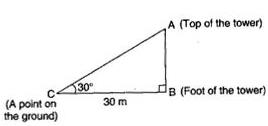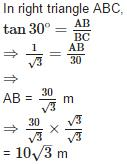Hence the height of the tower is 10√3 meters.

QUESTION: 2

### From a point on the ground which is 15m away from the foot of a tower, the angle of elevation is found to be 60°. The height of the tower is

Solution: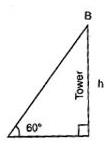Let the height of the tower be h meters.
In triangle AOB tan 60° = AB/OA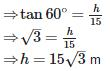Therfore the height of the tower is 15√3 meters

QUESTION: 3

### From a point P on the level ground, the angle of elevation of the top of a tower is 30°. If the tower is 100m high, the distance between P and the foot of the tower is

Solution: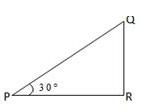Let QR be the height of the tower, then QR = 100 mQ And angle of elevation of the top of the tower be ∠PPR = 30°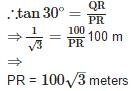Therefore, the distance between P and the foot of the tower is 100√3 metres.

QUESTION: 4

If the angle of depression of an object from a 75m high tower is 30°, then the distance of the object from the tower is

Solution:

In triangle ABC,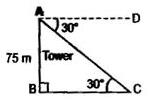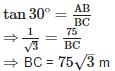Therefore, the distance between P and the foot of the tower is 75√3 metres.

QUESTION: 5

A ladder 14m long rests against a wall. If the foot of the ladder is 7m from the wall, then the angle of elevation is

Solution:

Let AC be the ladder of lenth 14 m and BC = 7 m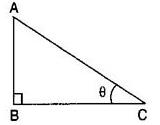Let angle of elevation ∠ACB = θ ∴ cos θ = BC/AC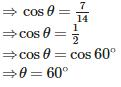QUESTION: 6

If the length of the shadow of a tower is √3 times that of its height, then the angle of elevation of the sun is

Solution:

Let AB be the tree and AP be the shadow.
Let AB = x meters. Then AP = x√3 meters
Also ∠APB = θ
In right angled triangle ABP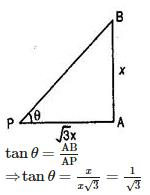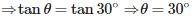Therfore the angle of elevation of the Sun is 30°.

QUESTION: 7

In a ΔABC right angled at B, ∠A = 30° and AC = 6cm, then the length of BC is

Solution: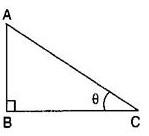In triangle ABC ∠A = 30°, and AC = 6 cm
Then lenth of BC,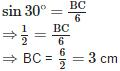Therfore the lenth of BC is 3 cm.

QUESTION: 8

If a kite is flying at a height of 10√3m from the level ground attached to a string inclined at 60° to the horizontal then the length of the string is

Solution:

Let AB be the length of the string and AC = 100 m
And ∠ABC = 60°
In triangle ABC,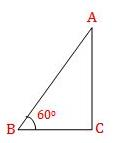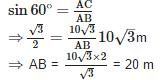Therefore, the length of the string is 20 m.

QUESTION: 9

The top of a broken tree has its top touching the ground at a distance of 10m from the bottom. If the angle made by the broken part with the ground is 30°, then the length of the broken part is

Solution: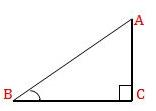Let AB be the broken part of the tree.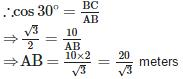Therefore, the length of the broken part of the tree is 20/√3 meters.

QUESTION: 10

An electric pole is 10√3 m high and its shadow is 10m in length, then the angle of elevation of the sun is

Solution: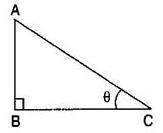Let AB be the electric pole of height 10√3 m and its shadow be BC of length 10 m And the angle of elevation of the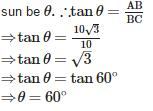QUESTION: 11

A kite is flying at a height of 60m from the level ground, attached to a string inclined at 30° to the horizontal. The length of the string is

Solution: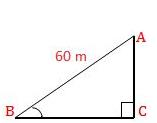Let kite is flying at a height AB = 60 m and angle of elevation = 30°
To find: Length of the string ACTherefore, the length of string is 120 m.  B C

QUESTION: 12

A circus artist is climbing a 20 m long rope, which is tightly stretched and tied from the top of a vertical pole to the ground. If the angle made by the rope with the ground level is 30°, then the height of the pole is

Solution:

In right triangle ABC,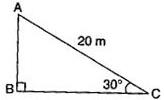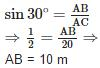Hence, the the height of the pole is 10 m.

QUESTION: 13

A river is 60m wide. A tree of unknown height is on one bank. The angle of elevation of the top of the tree from the point exactly opposite to the foot of the tree, on the other bank, is 30°. The height of the tree is

Solution:

Let the river BC be the width of 60 m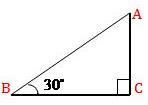and angle of elevation = 30°
To find: Height of the tree AC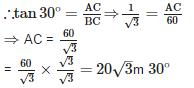Therefore, the height of the tree is 20 √3m.

QUESTION: 14

A bridge across a river makes an angle of 45° with the river bank. If the length of the bridge across the river is 200m, then the breadth of the river is

Solution:

Let the breath of the river be h meters.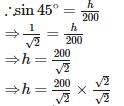= 100√2 meters Therefore, the breadth of river is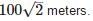QUESTION: 15

The upper part of a tree broken by the wind falls to the ground without being detached. The top of the broken part touches the ground at an angle of 30° at a point 8m from the foot of the tree. The original height of the tree is

Solution: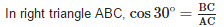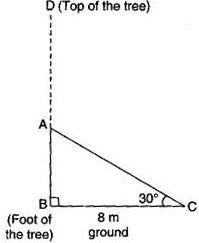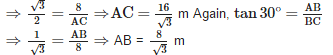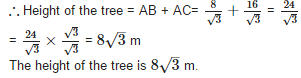QUESTION: 16

The angle of elevation of the top of a tower from two points P and Q at distances of ‘a’ and ‘b’ respectively from the base and in the same straight line with it are complementary. The height of the tower is

Solution:

Let TW be the tower of height hh meters and P and Q are two points such that PW = a and QW = b.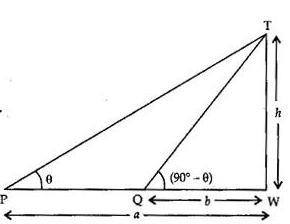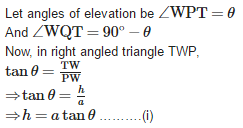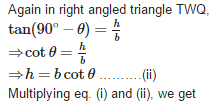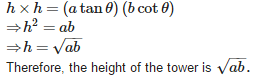QUESTION: 17

The tops of two poles of height 16m and 10m are connected by a wire. If the wire makes an angle of 60° with the horizontal, then the length of the wire is

Solution: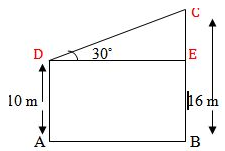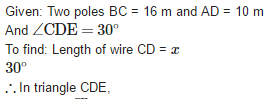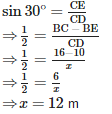Therefore, the length of the wire is 12 m.

QUESTION: 18

If the shadow of a tower is 30m long, when the sun’s elevation is 30°. The length of the shadow, when the sun’s elevation is 60°is

Solution: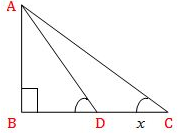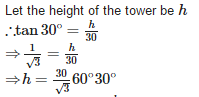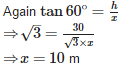Therefore, the length of the shadow is 10m long.

QUESTION: 19

If the angle of depression of a car from a 100m high tower is 45°, then the distance of the car from the tower is

Solution: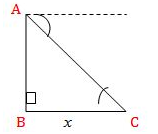Let the distance of the car from the tower be x meters.45°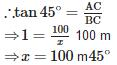Therefore, the distance of the car from the tower is 100 m.

QUESTION: 20

If the length of the shadow of a tower is equal to its height, then the angle of elevation of the sun is

Solution:

Let AB be the tower and AC be its shadow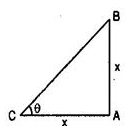And AB = AC= x m
Let the angle of elevation of the sun be θ.
Then ∠ACB = θ
In right angled triangle ABC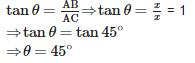Therefore, the angle of elevation of the Sun is 45°.

QUESTION: 21

If the height of the tower is √3 times of the length of its shadow, then the angle of elevation of the sun is

Solution: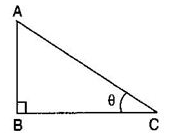Let the length of the shadow be x meters.
Then the height of the tower be √3x meter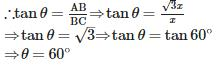QUESTION: 22

A ladder 12m long rests against a wall. If it reaches the wall at a height of 6√3m, then the angle of elevation is

Solution:
QUESTION: 23

A pole 10m high cast a shadow 10m long on the ground, then the sun’s elevation is

Solution: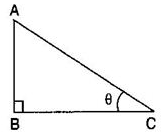Let the length of the shadow BC be 10 meters.
Then the height of the tower AB be 10 meter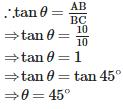QUESTION: 24

If altitude of the sun is 60°, the height of a tower which casts a shadow of length 30m is

Solution: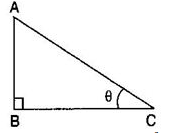Given: Angle of elevation θ = 60°
Let the height of the tower AB be hmeters.
And the length of the shadow BC is 30 meters.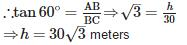Therefore the hieght of the tower is 30√3 meters.

QUESTION: 25

The measure of angle of elevation of the top of a tower 75√3m high from a point at a distance of 75m from the foot of the tower in a horizontal plane is

Solution:

Given: distance from a point to the foot of the tower = 75 m and the height of the tower = 75 m